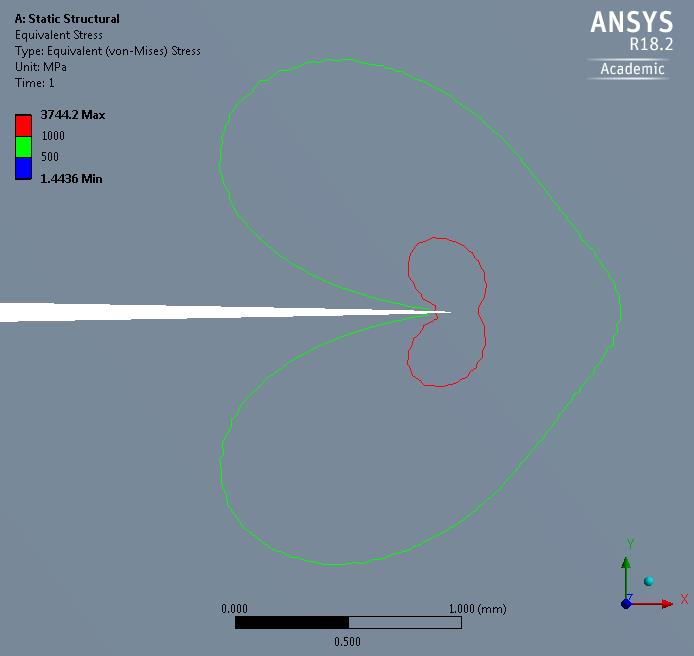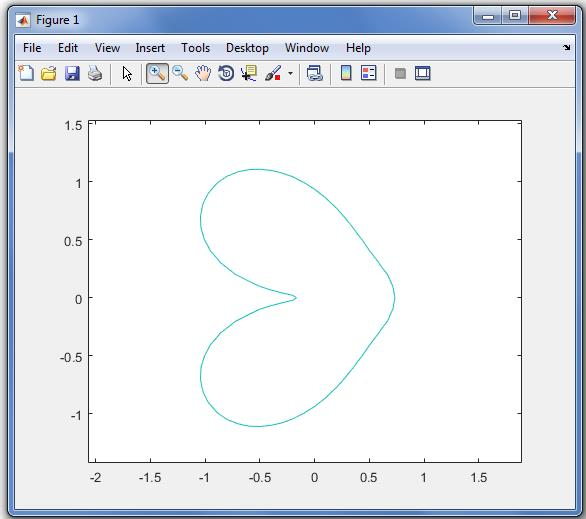peteroznewman
Subscriber

Subrat,

I made a similar model to yours but with a finer mesh and made this Isoline plotI followed Aniket's instructions and exported the stress data, and as I expected, ANSYS wrote the stress at each node, along with the x,y,z coordinates.

If you have matlab, you can import this data and with a few lines of matlab code, a grid of interpolated values can be created, then the matlab contour command can draw that same isoline that ANSYS plotted above.But matlab will list the x,y coordinates of this isoline, which is exactly what you wanted!

I don't understand why you wanted that, so if you care to explain, that would be interesting.